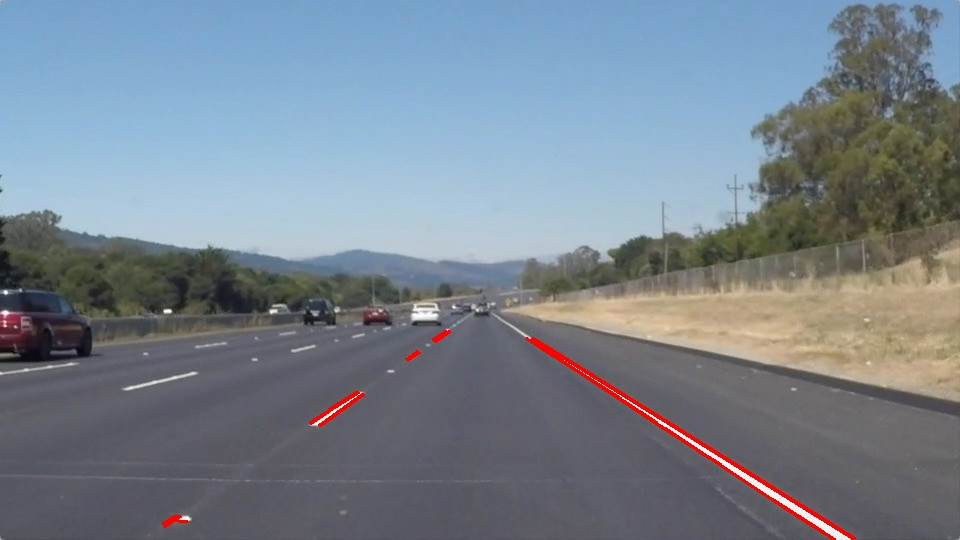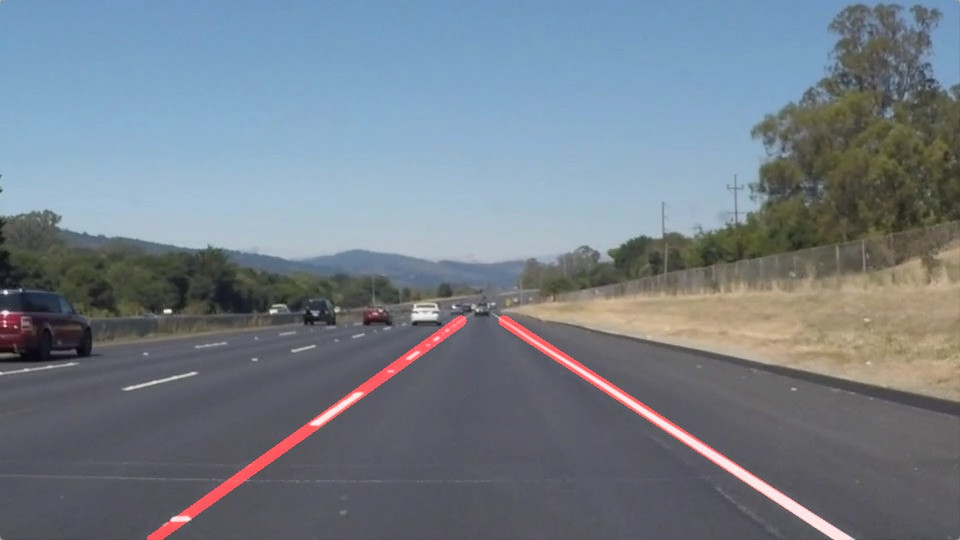#2019 iT 邦幫忙鐵人賽DAY 5
0
AI & Data

# 前言# 前階段準備流程

## Import Packages

``````#importing some useful packages
import matplotlib.pyplot as plt
import matplotlib.image as mpimg
import numpy as np
import cv2
%matplotlib inline
``````

``````image = mpimg.imread('test_images/solidWhiteRight.jpg')

#printing out some stats and plotting
print('This image is:', type(image), 'with dimensions:', image.shape)
plt.imshow(image)  # if you wanted to show a single color channel image called 'gray', for example, call as plt.imshow(gray, cmap='gray')
``````

## Helper Functions-實作 draw_lines函式

``````
import math

def grayscale(img):
"""Applies the Grayscale transform
This will return an image with only one color channel
but NOTE: to see the returned image as grayscale
(assuming your grayscaled image is called 'gray')
you should call plt.imshow(gray, cmap='gray')"""
return cv2.cvtColor(img, cv2.COLOR_RGB2GRAY)
# return cv2.cvtColor(img, cv2.COLOR_BGR2GRAY)
"""
canny algorithm:calculates the intensity gradients of the image and applies double threshold to determine edges.
"""
def canny(img, low_threshold, high_threshold):
"""Applies the Canny transform"""
return cv2.Canny(img, low_threshold, high_threshold)

def gaussian_blur(img, kernel_size):
"""Applies a Gaussian Noise kernel"""
return cv2.GaussianBlur(img, (kernel_size, kernel_size), 0)

def region_of_interest(img, vertices):
"""

Only keeps the region of the image defined by the polygon
formed from `vertices`. The rest of the image is set to black.
`vertices` should be a numpy array of integer points.
"""

#defining a 3 channel or 1 channel color to fill the mask with depending on the input image
if len(img.shape) > 2:
channel_count = img.shape  # i.e. 3 or 4 depending on your image
else:

#filling pixels inside the polygon defined by "vertices" with the fill color

#returning the image only where mask pixels are nonzero

def draw_lines(img, lines, color=[255, 0, 0], thickness=5):
"""
NOTE: this is the function you might want to use as a starting point once you want to
average/extrapolate the line segments you detect to map out the full
extent of the lane (going from the result shown in raw-lines-example.mp4
to that shown in P1_example.mp4).

Think about things like separating line segments by their
slope ((y2-y1)/(x2-x1)) to decide which segments are part of the left
line vs. the right line.  Then, you can average the position of each of
the lines and extrsfto the top and bottom of the lane.

This function draws `lines` with `color` and `thickness`.
Lines are drawn on the image inplace (mutates the image).
If you want to make the lines semi-transparent, think about combining
this function with the weighted_img() function below
"""
""" define the the top and bottom of the lane"""
top = 300
bottom =540
left_x1_set = []
left_y1_set = []
left_x2_set = []
left_y2_set = []
right_x1_set = []
right_y1_set = []
right_x2_set = []
right_y2_set = []

for line in lines:
for x1,y1,x2,y2 in line:
cv2.line(img, (x1, y1), (x2, y2), color, thickness)
slope = get_slope(x1,y1,x2,y2)
if slope < 0:
# Ignore obviously invalid lines
if slope > -.5 or slope < -.8:
continue
left_x1_set.append(x1)
left_y1_set.append(y1)
left_x2_set.append(x2)
left_y2_set.append(y2)
else:
# Ignore obviously invalid lines
if slope < .5 or slope > .8:
continue
right_x1_set.append(x1)
right_y1_set.append(y1)
right_x2_set.append(x2)
right_y2_set.append(y2)
try:
avg_right_x1 = int(np.mean(right_x1_set))
avg_right_y1 = int(np.mean(right_y1_set))
avg_right_x2 = int(np.mean(right_x2_set))
avg_right_y2 = int(np.mean(right_y2_set))
right_slope = get_slope(avg_right_x1,avg_right_y1,avg_right_x2,avg_right_y2)

right_y1 = top
right_x1 = int(avg_right_x1 + (right_y1 - avg_right_y1) / right_slope)
right_y2 = bottom
right_x2 = int(avg_right_x1 + (right_y2 - avg_right_y1) / right_slope)
cv2.line(img, (right_x1, right_y1), (right_x2, right_y2), color, thickness)
except ValueError:
# Don't error when a line cannot be drawn
pass

try:
avg_left_x1 = int(np.mean(left_x1_set))
avg_left_y1 = int(np.mean(left_y1_set))
avg_left_x2 = int(np.mean(left_x2_set))
avg_left_y2 = int(np.mean(left_y2_set))
left_slope = get_slope(avg_left_x1,avg_left_y1,avg_left_x2,avg_left_y2)

left_y1 = top
left_x1 = int(avg_left_x1 + (left_y1 - avg_left_y1) / left_slope)
left_y2 = bottom
left_x2 = int(avg_left_x1 + (left_y2 - avg_left_y1) / left_slope)
cv2.line(img, (left_x1, left_y1), (left_x2, left_y2), color, thickness)
except ValueError:
# Don't error when a line cannot be drawn
pass
def get_slope(x1,y1,x2,y2):
return ((y2-y1)/(x2-x1))

def hough_lines(img, rho, theta, threshold, min_line_len, max_line_gap):
"""
`img` should be the output of a Canny transform.

Returns an image with hough lines drawn.
"""
lines = cv2.HoughLinesP(img, rho, theta, threshold, np.array([]), minLineLength=min_line_len, maxLineGap=max_line_gap)
line_img = np.zeros((img.shape, img.shape, 3), dtype=np.uint8)
draw_lines(line_img, lines)
return line_img

# Python 3 has support for cool math symbols.
```python
def weighted_img(img, initial_img, α=0.8, β=1., γ=0.):
"""
`img` is the output of the hough_lines(), An image with lines drawn on it.
Should be a blank image (all black) with lines drawn on it.

`initial_img` should be the image before any processing.

The result image is computed as follows:

initial_img * α + img * β + γ
NOTE: initial_img and img must be the same shape!
"""
return cv2.addWeighted(initial_img, α, img, β, γ)
``````